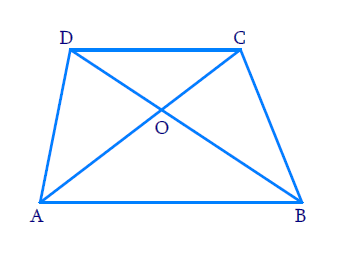# Ex.6.3 Q3 Triangles Solution - NCERT Maths Class 10

## Question

Diagonals $$AC$$ and $$BD$$ of a trapezium $$ABCD$$ with $$AB\, ||\, DC$$ intersect each other at the point $$O.$$ Using a similarity criterion for two triangles, show that \begin{align}\frac{OA}{OC}=\frac{OB}{OD}\end{align}.

DiagramVideo Solution
Triangles
Ex 6.3 | Question 3

## Text Solution

Reasoning:

If two angles of one triangle are respectively equal to the two angles of another triangle, then the two triangles are similar.

This is referred to as the $$AA$$ criterion.

Steps:

In $$\Delta \rm{A O B}, \Delta \rm{C O D}$$

\begin{align} & \angle AOB=\angle COD \\ & \text{(Vertically}\,\text{opposite}\,\,\text{angles)}\\ \\ & \angle BAO=\angle DCO \\ & (\text{Alternate interior angles }) \\\\ & \Rightarrow \Delta AOB\sim\Delta COD \\ & (\text{AA criterion}) \\ \end{align}

Hence \begin{align} \frac{O A}{O C}=\frac{O B}{O D}\end{align}

Learn from the best math teachers and top your exams

• Live one on one classroom and doubt clearing
• Practice worksheets in and after class for conceptual clarity
• Personalized curriculum to keep up with school